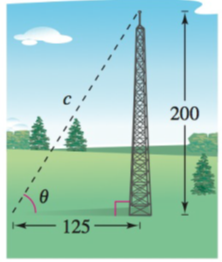Chapter 8.1, Problem 46E### Calculus: An Applied Approach (Min...

10th Edition
Ron Larson
ISBN: 9781305860919

#### Solutions

Chapter
Section### Calculus: An Applied Approach (Min...

10th Edition
Ron Larson
ISBN: 9781305860919
Textbook Problem
1 views

# Length A guy wire is stretched from a broadcasting tower at a point 200 feet above the ground to an anchor 125 feet from the base (see figure). How long is the wire?To determine

To calculate: The length of wire c if the wire is stretched from a 200 feet high broadcasting tower to an anchor on the ground which is at a distance of 125 feet from the tower.

Explanation

Given Information:

The wire is stretched from a 200 feet high broadcasting tower to an anchor on the ground which is at a distance of 125 feet from the tower.

The figure provide below shows the broadcasting tower.

Formula used:

Pythagorean theorem for any right triangle is such that c2=a2+b2, where a, b are the two perpendicular sides of a triangle and c is the length of hypotenuse.

Calculation:

Consider the length of the base be b, height be a and hypotenuse be c.

The height of the triangle a is 200 ft, the base b is 125 ft and the length of wire is c

### Still sussing out bartleby?

Check out a sample textbook solution.

See a sample solution

#### The Solution to Your Study Problems

Bartleby provides explanations to thousands of textbook problems written by our experts, many with advanced degrees!

Get Started

#### Evaluate the expression sin Exercises 116. 323

Finite Mathematics and Applied Calculus (MindTap Course List)

#### In Exercises 2340, find the indicated limit. 35. limx2x+2

Applied Calculus for the Managerial, Life, and Social Sciences: A Brief Approach

#### In problems 23-58, perform the indicated operations and simplify. 35.

Mathematical Applications for the Management, Life, and Social Sciences

#### Divide: +273

Elementary Technical Mathematics

#### For f(x) = tanh1 2x, f(x) = a) 2(sech1 2x)2 b) 2(sech2 2x)1 c) 214x2 d) 114x2

Study Guide for Stewart's Single Variable Calculus: Early Transcendentals, 8th

#### Find the unit tangent vector for at t = –1.

Study Guide for Stewart's Multivariable Calculus, 8th